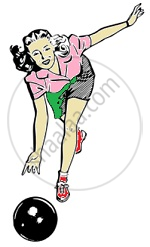Share

# Rolling Friction

## Rolling friction

• Force of friction which comes into play, between two surfaces, while one is rolling over the other is called rolling friction.

• Rolling friction is applicable for bodies whose point of contact keeps changing

• It is the force that opposes the motion of a body which is rolling over the surface of another

• Bowling balls, rotating wheels are examples illustrating Rolling friction

• Rolling friction is similar to kinetic friction.

So, fr= µr N
Here µr is the coefficient of rolling friction and N is the normal force.Coefficient of Rolling friction is lesser than that of Kinetic friction.
fr < fk < fs

S